# sbiofit

Perform nonlinear least-squares regression

Statistics and Machine Learning Toolbox™, Optimization Toolbox™, and Global Optimization Toolbox are recommended for this function.

## Syntax

``fitResults = sbiofit(sm,grpData,responseMap,estiminfo)``
``fitResults = sbiofit(sm,grpData,responseMap,estiminfo,dosing)``
``fitResults = sbiofit(sm,grpData,responseMap,estiminfo,dosing,functionName)``
``fitResults = sbiofit(sm,grpData,responseMap,estiminfo,dosing,functionName,options)``
``fitResults = sbiofit(sm,grpData,responseMap,estiminfo,dosing,functionName,options,variants)``
``fitResults = sbiofit(_,Name,Value)``
``````[fitResults,simdata] = sbiofit(_)``````

## Description

example

````fitResults = sbiofit(sm,grpData,responseMap,estiminfo)` estimates parameters of a SimBiology model `sm` using nonlinear least-squares regression.`grpData` is a `groupedData object` specifying the data to fit. `responseMap` defines the mapping between the model components and response data in `grpData`. `estimatedInfo` is an `EstimatedInfo object` that defines the estimated parameters in the model `sm`. `fitResults` is a `OptimResults object` or `NLINResults object` or a vector of these objects.`sbiofit` uses the first available estimation function among the following: `lsqnonlin` (Optimization Toolbox required), `nlinfit` (Statistics and Machine Learning Toolbox required), or `fminsearch`.By default, each group in `grpData` is fit separately, resulting in group-specific parameter estimates. If the model contains active doses and variants, they are applied before the simulation.```

example

````fitResults = sbiofit(sm,grpData,responseMap,estiminfo,dosing)` uses the dosing information specified by a matrix of SimBiology dose objects `dosing` instead of using the active doses of the model `sm` if there is any.```

example

````fitResults = sbiofit(sm,grpData,responseMap,estiminfo,dosing,functionName)` uses the estimation function specified by `functionName`. If the specified function is unavailable, a warning is issued and the first available default function is used.```

example

````fitResults = sbiofit(sm,grpData,responseMap,estiminfo,dosing,functionName,options)` uses the additional options specified by `options` for the function `functionName`.```

example

````fitResults = sbiofit(sm,grpData,responseMap,estiminfo,dosing,functionName,options,variants)` applies variant objects specified as `variants` instead of using any active variants of the model.```

example

````fitResults = sbiofit(_,Name,Value)` uses additional options specified by one or more `Name,Value` pair arguments.```

example

``````[fitResults,simdata] = sbiofit(_)``` also returns a vector of SimData objects `simdata` using any of the input arguments in the previous syntaxes. Note`sbiofit` unifies `sbionlinfit` and `sbioparamestim` estimation functions. Use `sbiofit` to perform nonlinear least-squares regression.`sbiofit` simulates the model using a `SimFunction object`, which automatically accelerates simulations by default. Hence it is not necessary to run `sbioaccelerate` before you call `sbiofit`. ```

## Examples

collapse all

Background

This example shows how to fit an individual's PK profile data to one-compartment model and estimate pharmacokinetic parameters.

Suppose you have drug plasma concentration data from an individual and want to estimate the volume of the central compartment and the clearance. Assume the drug concentration versus the time profile follows the monoexponential decline ${C}_{t}={C}_{0}{e}^{-{k}_{e}t}$, where ${C}_{t}$ is the drug concentration at time t, ${C}_{0}$ is the initial concentration, and ${k}_{e}$ is the elimination rate constant that depends on the clearance and volume of the central compartment ${k}_{e}=Cl/V$.

The synthetic data in this example was generated using the following model and parameters:

• One-compartment model with bolus dosing and first-order elimination

• Volume of the central compartment (`Central`) = 1.70 liter

• Clearance parameter (`Cl_Central`) = 0.55 liter/hour

• Constant error model

The data is stored as a table with variables `Time` and `Conc` that represent the time course of the plasma concentration of an individual after an intravenous bolus administration measured at 13 different time points. The variable units for `Time` and `Conc` are hour and milligram/liter, respectively.

```clear all load('data15.mat')```
```plot(data.Time,data.Conc,'b+') xlabel('Time (hour)'); ylabel('Drug Concentration (milligram/liter)');```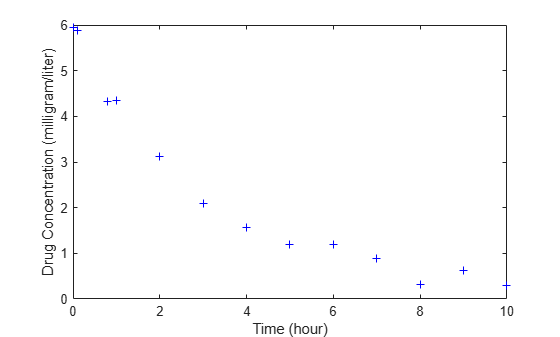Convert to groupedData Format

Convert the data set to a `groupedData` object, which is the required data format for the fitting function `sbiofit` for later use. A `groupedData` object also lets you set independent variable and group variable names (if they exist). Set the units of the `Time` and `Conc` variables. The units are optional and only required for the `UnitConversion` feature, which automatically converts matching physical quantities to one consistent unit system.

```gData = groupedData(data); gData.Properties.VariableUnits = {'hour','milligram/liter'}; gData.Properties```
```ans = struct with fields: Description: '' UserData: [] DimensionNames: {'Row' 'Variables'} VariableNames: {'Time' 'Conc'} VariableDescriptions: {} VariableUnits: {'hour' 'milligram/liter'} VariableContinuity: [] RowNames: {} CustomProperties: [1x1 matlab.tabular.CustomProperties] GroupVariableName: '' IndependentVariableName: 'Time' ```

`groupedData` automatically set the name of the `IndependentVariableName` property to the `Time` variable of the data.

Construct a One-Compartment Model

Use the built-in PK library to construct a one-compartment model with bolus dosing and first-order elimination where the elimination rate depends on the clearance and volume of the central compartment. Use the `configset` object to turn on unit conversion.

```pkmd = PKModelDesign; pkc1 = addCompartment(pkmd,'Central'); pkc1.DosingType = 'Bolus'; pkc1.EliminationType = 'linear-clearance'; pkc1.HasResponseVariable = true; model = construct(pkmd); configset = getconfigset(model); configset.CompileOptions.UnitConversion = true;```

For details on creating compartmental PK models using the SimBiology® built-in library, see Create Pharmacokinetic Models.

Define Dosing

Define a single bolus dose of 10 milligram given at time = 0. For details on setting up different dosing schedules, see Doses in SimBiology Models.

```dose = sbiodose('dose'); dose.TargetName = 'Drug_Central'; dose.StartTime = 0; dose.Amount = 10; dose.AmountUnits = 'milligram'; dose.TimeUnits = 'hour';```

Map Response Data to the Corresponding Model Component

The data contains drug concentration data stored in the `Conc` variable. This data corresponds to the `Drug_Central` species in the model. Therefore, map the data to `Drug_Central` as follows.

`responseMap = {'Drug_Central = Conc'};`

Specify Parameters to Estimate

The parameters to fit in this model are the volume of the central compartment (Central) and the clearance rate (Cl_Central). In this case, specify log-transformation for these biological parameters since they are constrained to be positive. The `estimatedInfo` object lets you specify parameter transforms, initial values, and parameter bounds if needed.

```paramsToEstimate = {'log(Central)','log(Cl_Central)'}; estimatedParams = estimatedInfo(paramsToEstimate,'InitialValue',[1 1],'Bounds',[1 5;0.5 2]);```

Estimate Parameters

Now that you have defined one-compartment model, data to fit, mapped response data, parameters to estimate, and dosing, use `sbiofit` to estimate parameters. The default estimation function that `sbiofit` uses will change depending on which toolboxes are available. To see which function was used during fitting, check the `EstimationFunction` property of the corresponding results object.

`fitConst = sbiofit(model,gData,responseMap,estimatedParams,dose);`

Display Estimated Parameters and Plot Results

Notice the parameter estimates were not far off from the true values (1.70 and 0.55) that were used to generate the data. You may also try different error models to see if they could further improve the parameter estimates.

`fitConst.ParameterEstimates`
```ans=2×4 table Name Estimate StandardError Bounds ______________ ________ _____________ __________ {'Central' } 1.6993 0.034821 1 5 {'Cl_Central'} 0.53358 0.01968 0.5 2 ```
```s.Labels.XLabel = 'Time (hour)'; s.Labels.YLabel = 'Concentration (milligram/liter)'; plot(fitConst,'AxesStyle',s);```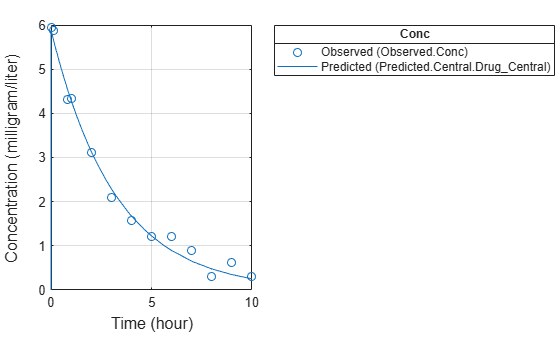Use Different Error Models

Try three other supported error models (proportional, combination of constant and proportional error models, and exponential).

```fitProp = sbiofit(model,gData,responseMap,estimatedParams,dose,... 'ErrorModel','proportional'); fitExp = sbiofit(model,gData,responseMap,estimatedParams,dose,... 'ErrorModel','exponential'); fitComb = sbiofit(model,gData,responseMap,estimatedParams,dose,... 'ErrorModel','combined');```

Use Weights Instead of an Error Model

You can specify weights as a numeric matrix, where the number of columns corresponds to the number of responses. Setting all weights to 1 is equivalent to the constant error model.

```weightsNumeric = ones(size(gData.Conc)); fitWeightsNumeric = sbiofit(model,gData,responseMap,estimatedParams,dose,'Weights',weightsNumeric);```

Alternatively, you can use a function handle that accepts a vector of predicted response values and returns a vector of weights. In this example, use a function handle that is equivalent to the proportional error model.

```weightsFunction = @(y) 1./y.^2; fitWeightsFunction = sbiofit(model,gData,responseMap,estimatedParams,dose,'Weights',weightsFunction);```

Compare Information Criteria for Model Selection

Compare the loglikelihood, AIC, and BIC values of each model to see which error model best fits the data. A larger likelihood value indicates the corresponding model fits the model better. For AIC and BIC, the smaller values are better.

```allResults = [fitConst,fitWeightsNumeric,fitWeightsFunction,fitProp,fitExp,fitComb]; errorModelNames = {'constant error model','equal weights','proportional weights', ... 'proportional error model','exponential error model',... 'combined error model'}; LogLikelihood = [allResults.LogLikelihood]'; AIC = [allResults.AIC]'; BIC = [allResults.BIC]'; t = table(LogLikelihood,AIC,BIC); t.Properties.RowNames = errorModelNames; t```
```t=6×3 table LogLikelihood AIC BIC _____________ _______ _______ constant error model 3.9866 -3.9732 -2.8433 equal weights 3.9866 -3.9732 -2.8433 proportional weights -3.8472 11.694 12.824 proportional error model -3.8257 11.651 12.781 exponential error model 1.1984 1.6032 2.7331 combined error model 3.9163 -3.8326 -2.7027 ```

Based on the information criteria, the constant error model (or equal weights) fits the data best since it has the largest loglikelihood value and the smallest AIC and BIC.

Display Estimated Parameter Values

Show the estimated parameter values of each model.

```Estimated_Central = zeros(6,1); Estimated_Cl_Central = zeros(6,1); t2 = table(Estimated_Central,Estimated_Cl_Central); t2.Properties.RowNames = errorModelNames; for i = 1:height(t2) t2{i,1} = allResults(i).ParameterEstimates.Estimate(1); t2{i,2} = allResults(i).ParameterEstimates.Estimate(2); end t2```
```t2=6×2 table Estimated_Central Estimated_Cl_Central _________________ ____________________ constant error model 1.6993 0.53358 equal weights 1.6993 0.53358 proportional weights 1.9045 0.51734 proportional error model 1.8777 0.51147 exponential error model 1.7872 0.51701 combined error model 1.7008 0.53271 ```

Conclusion

This example showed how to estimate PK parameters, namely the volume of the central compartment and clearance parameter of an individual, by fitting the PK profile data to one-compartment model. You compared the information criteria of each model and estimated parameter values of different error models to see which model best explained the data. Final fitted results suggested both the constant and combined error models provided the closest estimates to the parameter values used to generate the data. However, the constant error model is a better model as indicated by the loglikelihood, AIC, and BIC information criteria.

Suppose you have drug plasma concentration data from three individuals that you want to use to estimate corresponding pharmacokinetic parameters, namely the volume of central and peripheral compartment (`Central`, `Peripheral`), the clearance rate (`Cl_Central`), and intercompartmental clearance (`Q12`). Assume the drug concentration versus the time profile follows the biexponential decline ${C}_{t}=A{e}^{-at}+B{e}^{-bt}$, where Ct is the drug concentration at time t, and a and b are slopes for corresponding exponential declines.

The synthetic data set contains drug plasma concentration data measured in both central and peripheral compartments. The data was generated using a two-compartment model with an infusion dose and first-order elimination. These parameters were used for each individual.

`Central``Peripheral``Q12``Cl_Central`
Individual 11.900.680.240.57
Individual 22.106.050.360.95
Individual 31.704.210.460.95

The data is stored as a table with variables `ID`, `Time`, `CentralConc`, and `PeripheralConc`. It represents the time course of plasma concentrations measured at eight different time points for both central and peripheral compartments after an infusion dose.

```clear all load('data10_32R.mat') ```

Convert the data set to a `groupedData` object which is the required data format for the fitting function `sbiofit` for later use. A `groupedData` object also lets you set independent variable and group variable names (if they exist). Set the units of the `ID`, `Time`, `CentralConc`, and `PeripheralConc` variables. The units are optional and only required for the `UnitConversion` feature, which automatically converts matching physical quantities to one consistent unit system.

```gData = groupedData(data); gData.Properties.VariableUnits = {'','hour','milligram/liter','milligram/liter'}; gData.Properties ```
```ans = struct with fields: Description: '' UserData: [] DimensionNames: {'Row' 'Variables'} VariableNames: {'ID' 'Time' 'CentralConc' 'PeripheralConc'} VariableDescriptions: {} VariableUnits: {1x4 cell} VariableContinuity: [] RowNames: {} CustomProperties: [1x1 matlab.tabular.CustomProperties] GroupVariableName: 'ID' IndependentVariableName: 'Time' ```

Create a trellis plot that shows the PK profiles of three individuals.

```sbiotrellis(gData,'ID','Time',{'CentralConc','PeripheralConc'},... 'Marker','+','LineStyle','none'); ```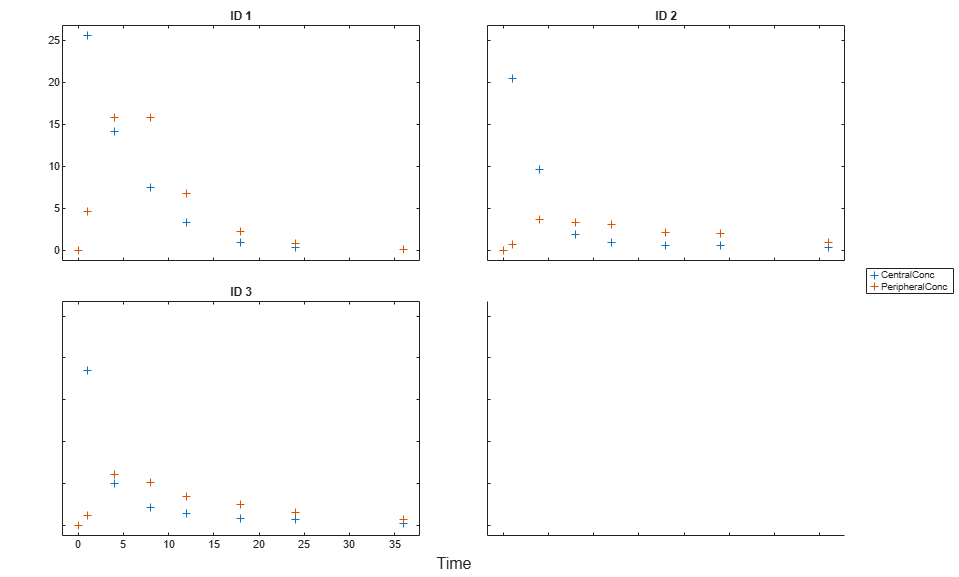Use the built-in PK library to construct a two-compartment model with infusion dosing and first-order elimination where the elimination rate depends on the clearance and volume of the central compartment. Use the configset object to turn on unit conversion.

```pkmd = PKModelDesign; pkc1 = addCompartment(pkmd,'Central'); pkc1.DosingType = 'Infusion'; pkc1.EliminationType = 'linear-clearance'; pkc1.HasResponseVariable = true; pkc2 = addCompartment(pkmd,'Peripheral'); model = construct(pkmd); configset = getconfigset(model); configset.CompileOptions.UnitConversion = true; ```

Assume every individual receives an infusion dose at time = 0, with a total infusion amount of 100 mg at a rate of 50 mg/hour. For details on setting up different dosing strategies, see Doses in SimBiology Models.

```dose = sbiodose('dose','TargetName','Drug_Central'); dose.StartTime = 0; dose.Amount = 100; dose.Rate = 50; dose.AmountUnits = 'milligram'; dose.TimeUnits = 'hour'; dose.RateUnits = 'milligram/hour'; ```

The data contains measured plasma concentrations in the central and peripheral compartments. Map these variables to the appropriate model species, which are `Drug_Central` and `Drug_Peripheral`.

```responseMap = {'Drug_Central = CentralConc','Drug_Peripheral = PeripheralConc'}; ```

The parameters to estimate in this model are the volumes of central and peripheral compartments (`Central` and `Peripheral`), intercompartmental clearance `Q12`, and clearance rate `Cl_Central`. In this case, specify log-transform for `Central` and `Peripheral` since they are constrained to be positive. The `estimatedInfo` object lets you specify parameter transforms, initial values, and parameter bounds (optional).

```paramsToEstimate = {'log(Central)','log(Peripheral)','Q12','Cl_Central'}; estimatedParam = estimatedInfo(paramsToEstimate,'InitialValue',[1 1 1 1]); ```

Fit the model to all of the data pooled together, that is, estimate one set of parameters for all individuals. The default estimation method that `sbiofit` uses will change depending on which toolboxes are available. To see which estimation function `sbiofit` used for the fitting, check the `EstimationFunction` property of the corresponding results object.

```pooledFit = sbiofit(model,gData,responseMap,estimatedParam,dose,'Pooled',true) ```
```pooledFit = OptimResults with properties: ExitFlag: 3 Output: [1x1 struct] GroupName: [] Beta: [4x3 table] ParameterEstimates: [4x3 table] J: [24x4x2 double] COVB: [4x4 double] CovarianceMatrix: [4x4 double] R: [24x2 double] MSE: 6.6220 SSE: 291.3688 Weights: [] LogLikelihood: -111.3904 AIC: 230.7808 BIC: 238.2656 DFE: 44 DependentFiles: {1x3 cell} EstimatedParameterNames: {'Central' 'Peripheral' 'Q12' 'Cl_Central'} ErrorModelInfo: [1x3 table] EstimationFunction: 'lsqnonlin' ```

Plot the fitted results versus the original data. Although three separate plots were generated, the data was fitted using the same set of parameters (that is, all three individuals had the same fitted line).

```plot(pooledFit); ```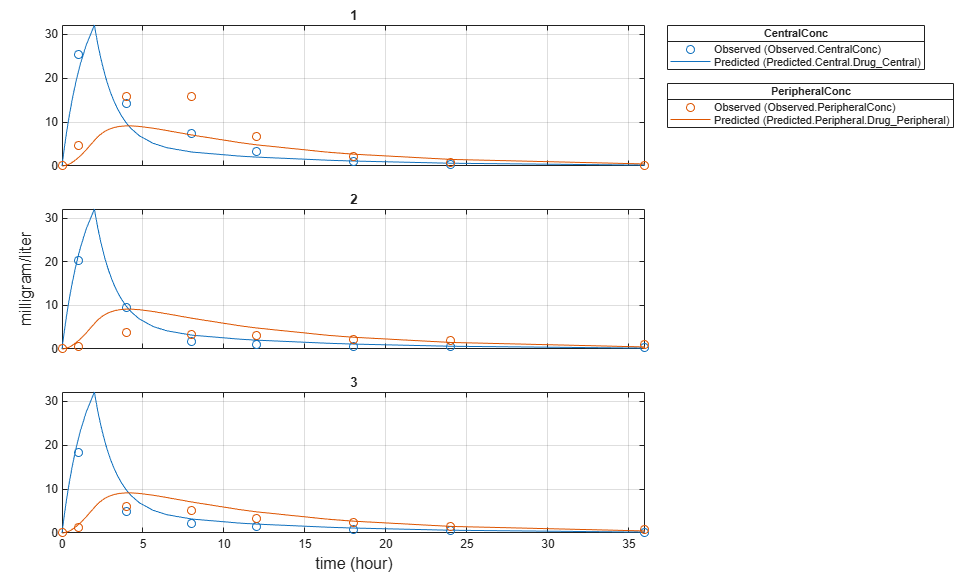Estimate one set of parameters for each individual and see if there is any improvement in the parameter estimates. In this example, since there are three individuals, three sets of parameters are estimated.

```unpooledFit = sbiofit(model,gData,responseMap,estimatedParam,dose,'Pooled',false); ```

Plot the fitted results versus the original data. Each individual was fitted differently (that is, each fitted line is unique to each individual) and each line appeared to fit well to individual data.

```plot(unpooledFit); ```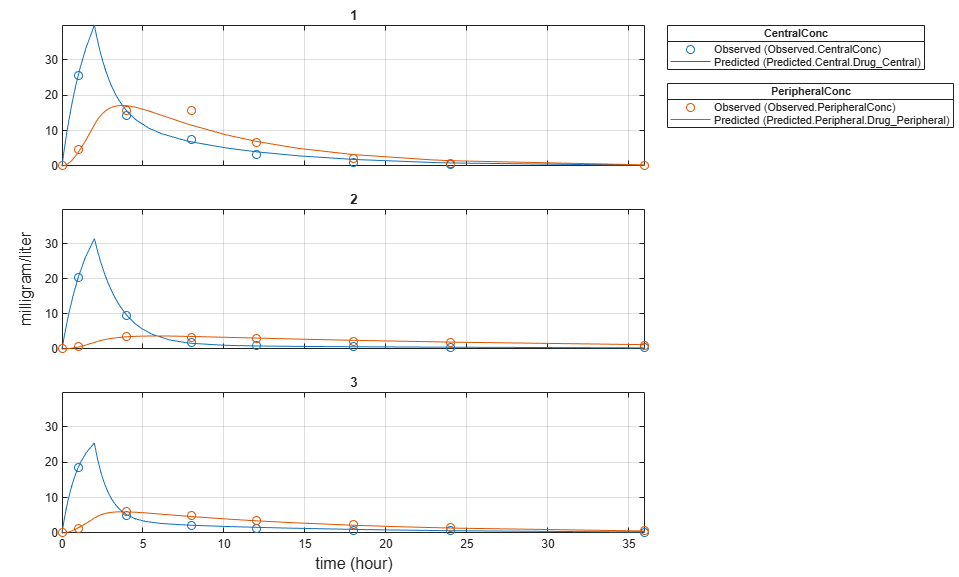Display the fitted results of the first individual. The MSE was lower than that of the pooled fit. This is also true for the other two individuals.

```unpooledFit(1) ```
```ans = OptimResults with properties: ExitFlag: 3 Output: [1x1 struct] GroupName: 1 Beta: [4x3 table] ParameterEstimates: [4x3 table] J: [8x4x2 double] COVB: [4x4 double] CovarianceMatrix: [4x4 double] R: [8x2 double] MSE: 2.1380 SSE: 25.6559 Weights: [] LogLikelihood: -26.4805 AIC: 60.9610 BIC: 64.0514 DFE: 12 DependentFiles: {1x3 cell} EstimatedParameterNames: {'Central' 'Peripheral' 'Q12' 'Cl_Central'} ErrorModelInfo: [1x3 table] EstimationFunction: 'lsqnonlin' ```

Generate a plot of the residuals over time to compare the pooled and unpooled fit results. The figure indicates unpooled fit residuals are smaller than those of pooled fit as expected. In addition to comparing residuals, other rigorous criteria can be used to compare the fitted results.

```t = [gData.Time;gData.Time]; res_pooled = vertcat(pooledFit.R); res_pooled = res_pooled(:); res_unpooled = vertcat(unpooledFit.R); res_unpooled = res_unpooled(:); plot(t,res_pooled,'o','MarkerFaceColor','w','markerEdgeColor','b') hold on plot(t,res_unpooled,'o','MarkerFaceColor','b','markerEdgeColor','b') refl = refline(0,0); % A reference line representing a zero residual title('Residuals versus Time'); xlabel('Time'); ylabel('Residuals'); legend({'Pooled','Unpooled'}); ```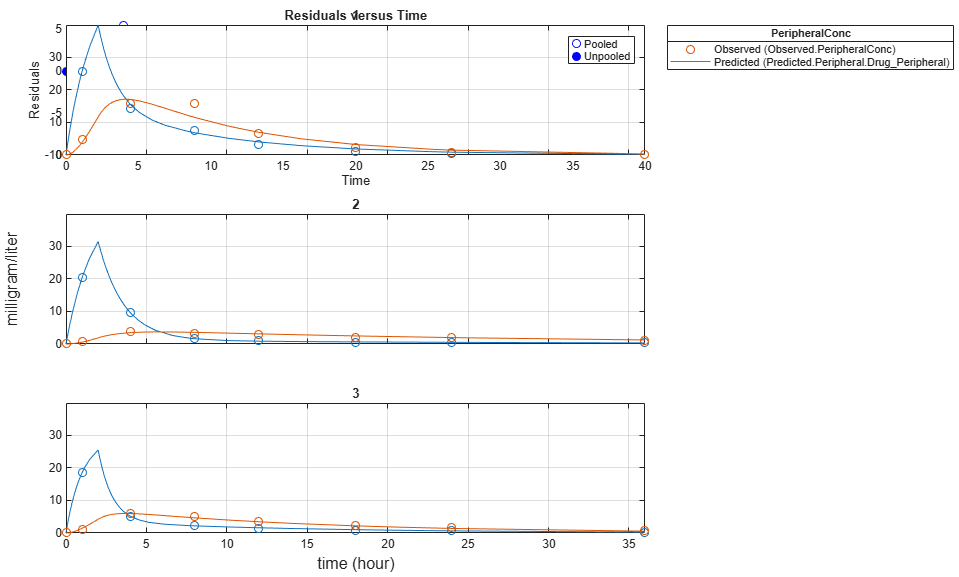This example showed how to perform pooled and unpooled estimations using `sbiofit`. As illustrated, the unpooled fit accounts for variations due to the specific subjects in the study, and, in this case, the model fits better to the data. However, the pooled fit returns population-wide parameters. If you want to estimate population-wide parameters while considering individual variations, use `sbiofitmixed`.

This example shows how to estimate category-specific (such as young versus old, male versus female), individual-specific, and population-wide parameters using PK profile data from multiple individuals.

Background

Suppose you have drug plasma concentration data from 30 individuals and want to estimate pharmacokinetic parameters, namely the volumes of central and peripheral compartment, the clearance, and intercompartmental clearance. Assume the drug concentration versus the time profile follows the biexponential decline ${C}_{t}=A{e}^{-at}+B{e}^{-bt}$, where ${C}_{t}$ is the drug concentration at time t, and $a$ and $b$ are slopes for corresponding exponential declines.

This synthetic data contains the time course of plasma concentrations of 30 individuals after a bolus dose (100 mg) measured at different times for both central and peripheral compartments. It also contains categorical variables, namely `Sex` and `Age`.

```clear load('sd5_302RAgeSex.mat')```

Convert to groupedData Format

Convert the data set to a `groupedData` object, which is the required data format for the fitting function `sbiofit`. A `groupedData` object also allows you set independent variable and group variable names (if they exist). Set the units of the `ID`, `Time`, `CentralConc`, `PeripheralConc`, `Age`, and `Sex` variables. The units are optional and only required for the `UnitConversion` feature, which automatically converts matching physical quantities to one consistent unit system.

```gData = groupedData(data); gData.Properties.VariableUnits = {'','hour','milligram/liter','milligram/liter','',''}; gData.Properties```
```ans = struct with fields: Description: '' UserData: [] DimensionNames: {'Row' 'Variables'} VariableNames: {1x6 cell} VariableDescriptions: {} VariableUnits: {1x6 cell} VariableContinuity: [] RowNames: {} CustomProperties: [1x1 matlab.tabular.CustomProperties] GroupVariableName: 'ID' IndependentVariableName: 'Time' ```

The `IndependentVariableName` and `GroupVariableName` properties have been automatically set to the `Time` and `ID` variables of the data.

Visualize Data

Display the response data for each individual.

```t = sbiotrellis(gData,'ID','Time',{'CentralConc','PeripheralConc'},... 'Marker','+','LineStyle','none'); % Resize the figure. t.hFig.Position(:) = [100 100 1280 800];```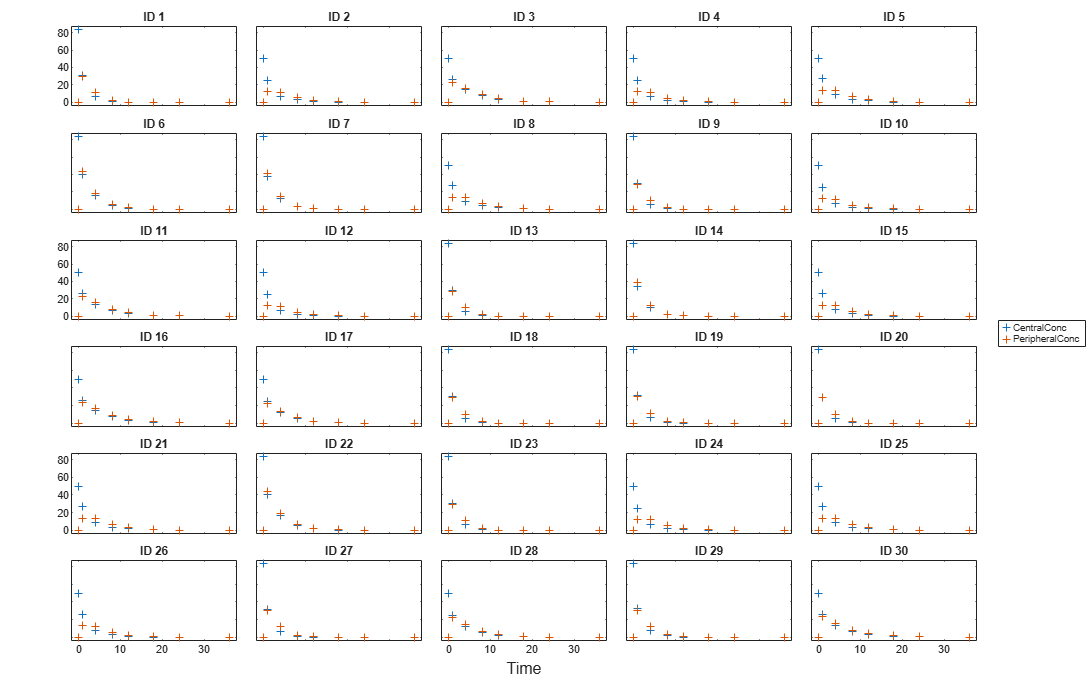Set Up a Two-Compartment Model

Use the built-in PK library to construct a two-compartment model with infusion dosing and first-order elimination where the elimination rate depends on the clearance and volume of the central compartment. Use the `configset` object to turn on unit conversion.

```pkmd = PKModelDesign; pkc1 = addCompartment(pkmd,'Central'); pkc1.DosingType = 'Bolus'; pkc1.EliminationType = 'linear-clearance'; pkc1.HasResponseVariable = true; pkc2 = addCompartment(pkmd,'Peripheral'); model = construct(pkmd); configset = getconfigset(model); configset.CompileOptions.UnitConversion = true;```

For details on creating compartmental PK models using the SimBiology® built-in library, see Create Pharmacokinetic Models.

Define Dosing

Assume every individual receives a bolus dose of 100 mg at time = 0. For details on setting up different dosing strategies, see Doses in SimBiology Models.

```dose = sbiodose('dose','TargetName','Drug_Central'); dose.StartTime = 0; dose.Amount = 100; dose.AmountUnits = 'milligram'; dose.TimeUnits = 'hour';```

Map the Response Data to Corresponding Model Components

The data contains measured plasma concentration in the central and peripheral compartments. Map these variables to the appropriate model components, which are `Drug_Central` and `Drug_Peripheral`.

`responseMap = {'Drug_Central = CentralConc','Drug_Peripheral = PeripheralConc'};`

Specify Parameters to Estimate

Specify the volumes of central and peripheral compartments `Central` and `Peripheral`, intercompartmental clearance `Q12`, and clearance `Cl_Central` as parameters to estimate. The `estimatedInfo` object lets you optionally specify parameter transforms, initial values, and parameter bounds. Since both `Central` and `Peripheral` are constrained to be positive, specify a log-transform for each parameter.

```paramsToEstimate = {'log(Central)', 'log(Peripheral)', 'Q12', 'Cl_Central'}; estimatedParam = estimatedInfo(paramsToEstimate,'InitialValue',[1 1 1 1]);```

Estimate Individual-Specific Parameters

Estimate one set of parameters for each individual by setting the `'Pooled'` name-value pair argument to `false`.

`unpooledFit = sbiofit(model,gData,responseMap,estimatedParam,dose,'Pooled',false);`

Display Results

Plot the fitted results versus the original data for each individual (group).

```t = plot(unpooledFit); % Resize the figure. t.hFig.Position(:) = [100 100 1280 800];```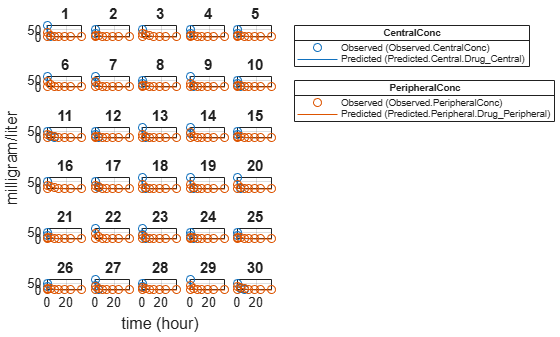For an unpooled fit, `sbiofit` always returns one results object for each individual.

Examine Parameter Estimates for Category Dependencies

Explore the unpooled estimates to see if there is any category-specific parameters, that is, if some parameters are related to one or more categories. If there are any category dependencies, it might be possible to reduce the number of degrees of freedom by estimating just category-specific values for those parameters.

First extract the ID and category values for each ID

`catParamValues = unique(gData(:,{'ID','Sex','Age'}));`

Add variables to the table containing each parameter's estimate.

```allParamValues = vertcat(unpooledFit.ParameterEstimates); catParamValues.Central = allParamValues.Estimate(strcmp(allParamValues.Name, 'Central')); catParamValues.Peripheral = allParamValues.Estimate(strcmp(allParamValues.Name, 'Peripheral')); catParamValues.Q12 = allParamValues.Estimate(strcmp(allParamValues.Name, 'Q12')); catParamValues.Cl_Central = allParamValues.Estimate(strcmp(allParamValues.Name, 'Cl_Central'));```

Plot estimates of each parameter for each category. `gscatter` requires Statistics and Machine Learning Toolbox™. If you do not have it, use other alternative plotting functions such as `plot`.

```h = figure; ylabels = {'Central','Peripheral','Cl\_Central','Q12'}; plotNumber = 1; for i = 1:4 thisParam = estimatedParam(i).Name; % Plot for Sex category subplot(4,2,plotNumber); plotNumber = plotNumber + 1; gscatter(double(catParamValues.Sex), catParamValues.(thisParam), catParamValues.Sex); ax = gca; ax.XTick = []; ylabel(ylabels(i)); legend('Location','bestoutside') % Plot for Age category subplot(4,2,plotNumber); plotNumber = plotNumber + 1; gscatter(double(catParamValues.Age), catParamValues.(thisParam), catParamValues.Age); ax = gca; ax.XTick = []; ylabel(ylabels(i)); legend('Location','bestoutside') end % Resize the figure. h.Position(:) = [100 100 1280 800];```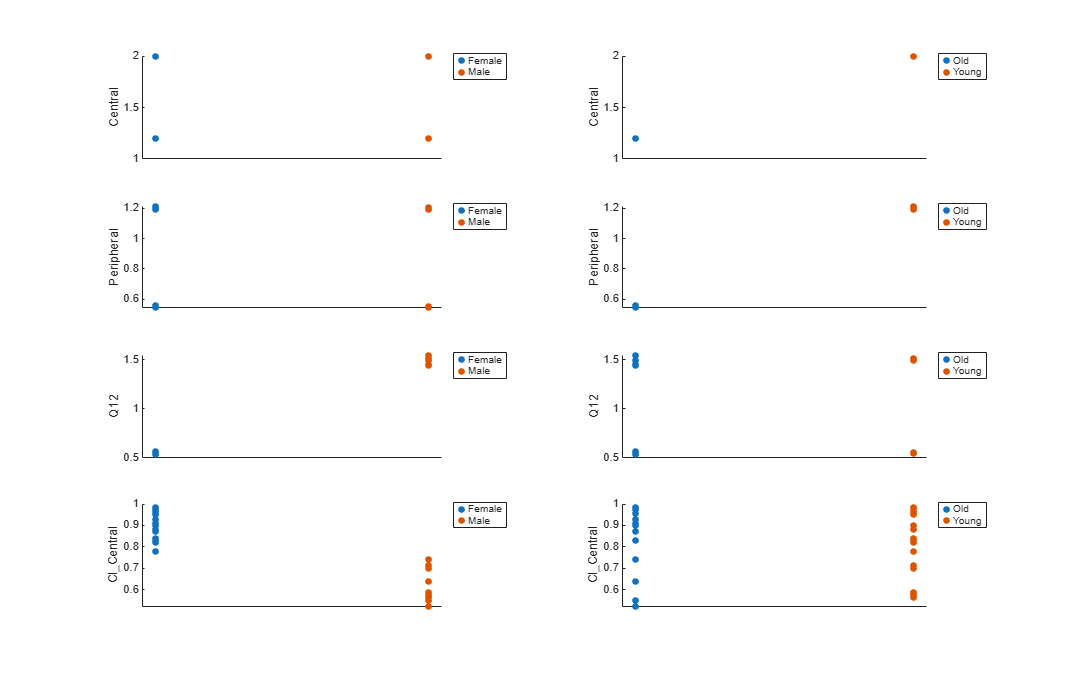Based on the plot, it seems that young individuals tend to have higher volumes of central and peripheral compartments (`Central`, `Peripheral`) than old individuals (that is, the volumes seem to be age-specific). In addition, males tend to have higher clearance rates (`Cl_Central`) than females but the opposite for the Q12 parameter (that is, the clearance and Q12 seem to be sex-specific).

Estimate Category-Specific Parameters

Use the `'CategoryVariableName'` property of the `estimatedInfo` object to specify which category to use during fitting. Use `'Sex'` as the group to fit for the clearance `Cl_Central` and `Q12` parameters. Use `'Age'` as the group to fit for the `Central` and `Peripheral` parameters.

```estimatedParam(1).CategoryVariableName = 'Age'; estimatedParam(2).CategoryVariableName = 'Age'; estimatedParam(3).CategoryVariableName = 'Sex'; estimatedParam(4).CategoryVariableName = 'Sex'; categoryFit = sbiofit(model,gData,responseMap,estimatedParam,dose)```
```categoryFit = OptimResults with properties: ExitFlag: 3 Output: [1x1 struct] GroupName: [] Beta: [8x5 table] ParameterEstimates: [120x6 table] J: [240x8x2 double] COVB: [8x8 double] CovarianceMatrix: [8x8 double] R: [240x2 double] MSE: 0.4362 SSE: 205.8690 Weights: [] LogLikelihood: -477.9195 AIC: 971.8390 BIC: 1.0052e+03 DFE: 472 DependentFiles: {1x3 cell} EstimatedParameterNames: {'Central' 'Peripheral' 'Q12' 'Cl_Central'} ErrorModelInfo: [1x3 table] EstimationFunction: 'lsqnonlin' ```

When fitting by category (or group), `sbiofit` always returns one results object, not one for each category level. This is because both male and female individuals are considered to be part of the same optimization using the same error model and error parameters, similarly for the young and old individuals.

Plot Results

Plot the category-specific estimated results.

```t = plot(categoryFit); % Resize the figure. t.hFig.Position(:) = [100 100 1280 800];```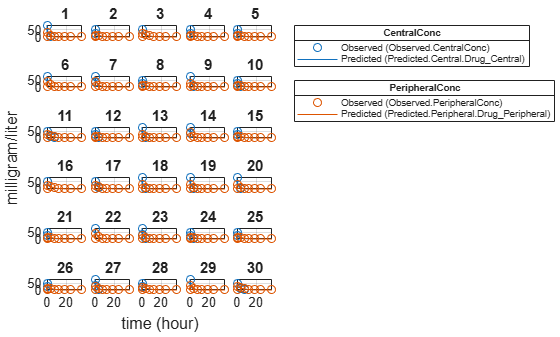For the `Cl_Central` and `Q12` parameters, all males had the same estimates, and similarly for the females. For the `Central` and `Peripheral` parameters, all young individuals had the same estimates, and similarly for the old individuals.

Estimate Population-Wide Parameters

To better compare the results, fit the model to all of the data pooled together, that is, estimate one set of parameters for all individuals by setting the `'Pooled'` name-value pair argument to `true`. The warning message tells you that this option will ignore any category-specific information (if they exist).

`pooledFit = sbiofit(model,gData,responseMap,estimatedParam,dose,'Pooled',true);`
```Warning: CategoryVariableName property of the estimatedInfo object is ignored when using the 'Pooled' option. ```

Plot Results

Plot the fitted results versus the original data. Although a separate plot was generated for each individual, the data was fitted using the same set of parameters (that is, all individuals had the same fitted line).

```t = plot(pooledFit); % Resize the figure. t.hFig.Position(:) = [100 100 1280 800];```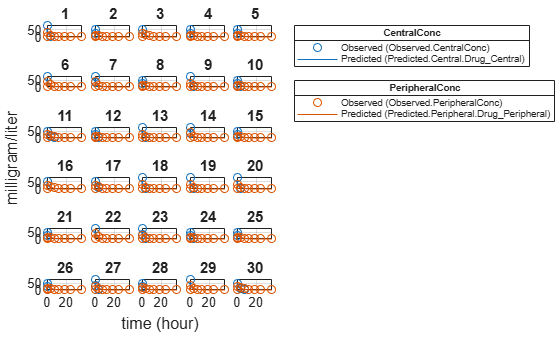Compare Residuals

Compare residuals of `CentralConc` and `PeripheralConc` responses for each fit.

```t = gData.Time; allResid(:,:,1) = pooledFit.R; allResid(:,:,2) = categoryFit.R; allResid(:,:,3) = vertcat(unpooledFit.R); h = figure; responseList = {'CentralConc', 'PeripheralConc'}; for i = 1:2 subplot(2,1,i); oneResid = squeeze(allResid(:,i,:)); plot(t,oneResid,'o'); refline(0,0); % A reference line representing a zero residual title(sprintf('Residuals (%s)', responseList{i})); xlabel('Time'); ylabel('Residuals'); legend({'Pooled','Category-Specific','Unpooled'}); end % Resize the figure. h.Position(:) = [100 100 1280 800];```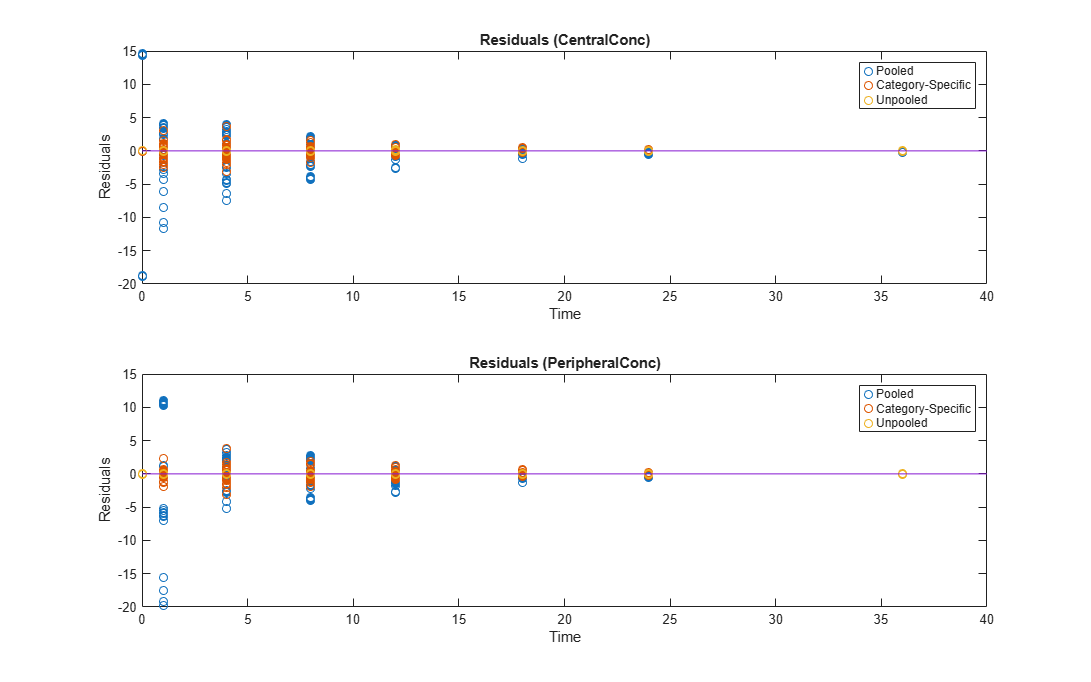As shown in the plot, the unpooled fit produced the best fit to the data as it fit the data to each individual. This was expected since it used the most number of degrees of freedom. The category-fit reduced the number of degrees of freedom by fitting the data to two categories (sex and age). As a result, the residuals were larger than the unpooled fit, but still smaller than the population-fit, which estimated just one set of parameters for all individuals. The category-fit might be a good compromise between the unpooled and pooled fitting provided that any hierarchical model exists within your data.

This example uses the yeast heterotrimeric G protein model and experimental data reported by . For details about the model, see the Background section in Parameter Scanning, Parameter Estimation, and Sensitivity Analysis in the Yeast Heterotrimeric G Protein Cycle.

`sbioloadproject gprotein`

Store the experimental data containing the time course for the fraction of active G protein.

```time = [0 10 30 60 110 210 300 450 600]'; GaFracExpt = [0 0.35 0.4 0.36 0.39 0.33 0.24 0.17 0.2]';```

Create a `groupedData` object based on the experimental data.

```tbl = table(time,GaFracExpt); grpData = groupedData(tbl);```

Map the appropriate model component to the experimental data. In other words, indicate which species in the model corresponds to which response variable in the data. In this example, map the model parameter `GaFrac` to the experimental data variable `GaFracExpt` from `grpData`.

`responseMap = 'GaFrac = GaFracExpt';`

Use an `estimatedInfo` object to define the model parameter `kGd` as a parameter to be estimated.

`estimatedParam = estimatedInfo('kGd');`

Perform the parameter estimation.

`fitResult = sbiofit(m1,grpData,responseMap,estimatedParam);`

View the estimated parameter value of `kGd`.

`fitResult.ParameterEstimates`
```ans=1×3 table Name Estimate StandardError _______ ________ _____________ {'kGd'} 0.11307 3.4439e-05 ```

Suppose you want to plot the model simulation results using the estimated parameter value. You can either rerun the `sbiofit` function and specify to return the optional second output argument, which contains simulation results, or use the `fitted` method to retrieve the results without rerunning `sbiofit`.

`[yfit,paramEstim] = fitted(fitResult);`

Plot the simulation results.

`sbioplot(yfit);`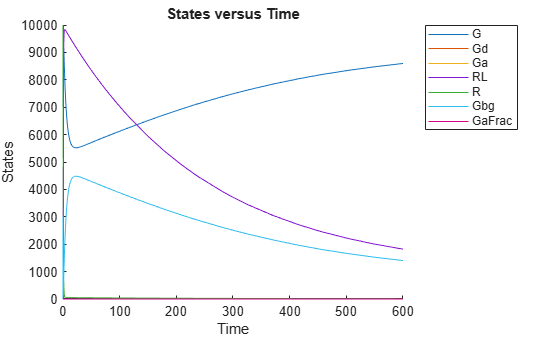This example shows how to estimate the time lag before a bolus dose was administered and the duration of the dose using a one-compartment model.

`load lagDurationData.mat`

Plot the data.

```plot(data.Time,data.Conc,'x') xlabel('Time (hour)') ylabel('Conc (milligram/liter)')```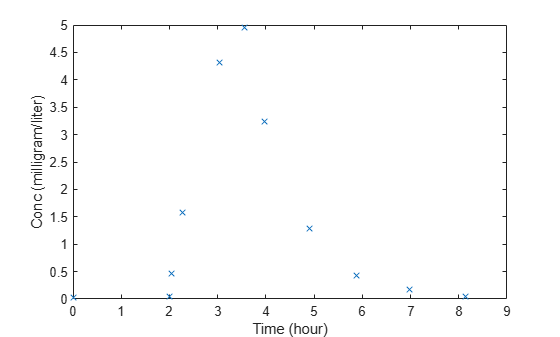Convert to groupedData.

```gData = groupedData(data); gData.Properties.VariableUnits = {'hour','milligram/liter'};```

Create a one-compartment model.

```pkmd = PKModelDesign; pkc1 = addCompartment(pkmd,'Central'); pkc1.DosingType = 'Bolus'; pkc1.EliminationType = 'linear-clearance'; pkc1.HasResponseVariable = true; model = construct(pkmd); configset = getconfigset(model); configset.CompileOptions.UnitConversion = true;```

Add two parameters that represent the time lag and duration of a dose. The lag parameter specifies the time lag before the dose is administered. The duration parameter specifies the length of time it takes to administer a dose.

```lagP = addparameter(model,'lagP'); lagP.ValueUnits = 'hour'; durP = addparameter(model,'durP'); durP.ValueUnits = 'hour';```

Create a dose object. Set the `LagParameterName` and `DurationParameterName` properties of the dose to the names of the lag and duration parameters, respectively.

```dose = sbiodose('dose'); dose.TargetName = 'Drug_Central'; dose.StartTime = 0; dose.Amount = 10; dose.AmountUnits = 'milligram'; dose.TimeUnits = 'hour'; dose.LagParameterName = 'lagP'; dose.DurationParameterName = 'durP';```

Map the model species to the corresponding data.

`responseMap = {'Drug_Central = Conc'};`

Specify the lag and duration parameters as parameters to estimate. Log-transform the parameters. Initialize them to 2 and set the upper bound and lower bound.

```paramsToEstimate = {'log(lagP)','log(durP)'}; estimatedParams = estimatedInfo(paramsToEstimate,'InitialValue',2,'Bounds',[1 5]);```

Perform parameter estimation.

`fitResults = sbiofit(model,gData,responseMap,estimatedParams,dose,'fminsearch')`
```fitResults = OptimResults with properties: ExitFlag: 1 Output: [1x1 struct] GroupName: One group Beta: [2x4 table] ParameterEstimates: [2x4 table] J: [11x2 double] COVB: [2x2 double] CovarianceMatrix: [2x2 double] R: [11x1 double] MSE: 0.0024 SSE: 0.0213 Weights: [] LogLikelihood: 18.7511 AIC: -33.5023 BIC: -32.7065 DFE: 9 DependentFiles: {1x2 cell} EstimatedParameterNames: {'lagP' 'durP'} ErrorModelInfo: [1x3 table] EstimationFunction: 'fminsearch' ```

Display the result.

`fitResults.ParameterEstimates`
```ans=2×4 table Name Estimate StandardError Bounds ________ ________ _____________ ______ {'lagP'} 1.986 0.0051568 1 5 {'durP'} 1.527 0.012956 1 5 ```
`plot(fitResults)`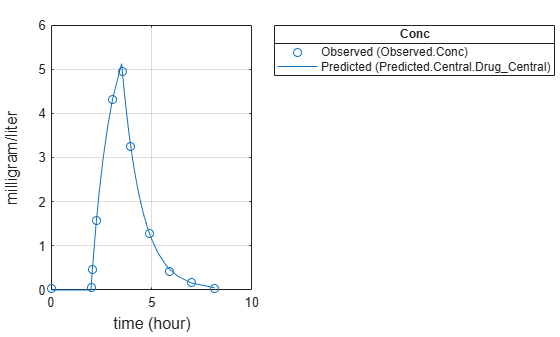## Input Arguments

collapse all

SimBiology model, specified as a SimBiology `model object`. The active `configset object` of the model contains solver settings for simulation. Any active doses and variants are applied to the model during simulation unless specified otherwise using the `dosing` and `variants` input arguments, respectively.

Data to fit, specified as a ```groupedData object```.

The name of the time variable must be defined in the `IndependentVariableName` property of `grpData`. For instance, if the time variable name is `'TIME'`, then specify it as follows.

`grpData.Properties.IndependentVariableName = 'TIME';`

If the data contains more than one group of measurements, the grouping variable name must be defined in the `GroupVariableName` property of `grpData`. For example, if the grouping variable name is `'GROUP'`, then specify it as follows.

`grpData.Properties.GroupVariableName = 'GROUP';`
A group usually refers to a set of measurements that represent a single time course, often corresponding to a particular individual, or experimental condition.

### Note

`sbiofit` uses the `categorical` function to identify groups. If any group values are converted to the same value by `categorical`, then those observations are treated as belonging to the same group. For instance, if some observations have no group information (that is, an empty character vector `''`), then `categorical` converts empty character vectors to `<undefined>`, and these observations are treated as one group.

Mapping information of model components to `grpData`, specified as a character vector, string, string vector, or cell array of character vectors.

Each character vector or string is an equation-like expression, similar to assignment rules in SimBiology. It contains the name (or qualified name) of a quantity (species, compartment, or parameter) or an `observable` object in the model `sm`, followed by the character `'='` and the name of a variable in `grpData`. For clarity, white spaces are allowed between names and `'='`.

For example, if you have the concentration data `'CONC'` in `grpData` for a species `'Drug_Central'`, you can specify it as follows.

`responseMap = 'Drug_Central = CONC';`

To name a species unambiguously, use the qualified name, which includes the name of the compartment. To name a reaction-scoped parameter, use the reaction name to qualify the parameter.

If the model component name or `grpData` variable name is not a valid MATLAB® variable name, surround it by square brackets, such as:

`responseMap = '[Central 1].Drug = [Central 1 Conc]';`

If a variable name itself contains square brackets, you cannot use it in the expression to define the mapping information.

An error is issued if any (qualified) name matches two components of the same type. However, you can use a (qualified) name that matches two components of different types, and the function first finds the species with the given name, followed by compartments and then parameters.

Estimated parameters, specified as an `EstimatedInfo object` or vector of `estimatedInfo` objects that defines the estimated parameters in the model `sm`, and other optional information such as their initial estimates, transformations, bound constraints, and categories. Supported transforms are `log`, `logit`, and `probit`.

You can specify bounds for all estimation methods. The lower bound must be less than the upper bound. For details, see boundValues.

When using `scattersearch`, you must specify finite transformed bounds for each estimated parameter.

When using `fminsearch`, `nlinfit`, or `fminunc` with bounds, the objective function returns `Inf` if bounds are exceeded. When you turn on options such as `FunValCheck`, the optimization might error if bounds are exceeded during estimation. If using `nlinfit`, it might report warnings about the Jacobian being ill-conditioned or not being able to estimate if the final result is too close to the bounds.

If you do not specify `Pooled` name-value pair argument, `sbiofit` uses `CategoryVariableName` property of `estiminfo` to decide if parameters must be estimated for each individual, group, category, or all individuals as a whole. Use the `Pooled` option to override any `CategoryVariableName` values. For details about `CategoryVariableName` property, see `EstimatedInfo object`.

### Note

`sbiofit` uses the `categorical` function to identify groups or categories. If any group values are converted to the same value by `categorical`, then those observations are treated as belonging to the same group. For instance, if some observations have no group information (that is, an empty character vector `''` as a group value), then `categorical` converts empty character vectors to `<undefined>`, and these observations are treated as one group.

Dosing information, specified as an empty array, or 2-D matrix of dose objects (`ScheduleDose object` or `RepeatDose object`).

• If empty, no doses are applied during simulation, even if the model has active doses.

• If not empty, the matrix must have a single row or one row per group in the input data. If it has a single row, same doses are applied to all groups during simulation. If it has multiple rows, each row is applied to a separate group, in the same order as the groups appear in the input data.

• Multiple columns are allowed so that you can apply multiple dose objects to each group. All doses in a column must reference the same components in the model. For example, doses in the same column must have the same dose target (`TargetName`). If you parameterize any property of a dose, then all doses within the column must have this property parameterized to the same parameter. If some groups require more doses than others, then fill in the matrix with default (dummy) doses.

• A default dose has default values for all properties, except for the `Name` property. Create a default dose as follows.

`d1 = sbiodose('d1');`

• In addition to manually constructing dose objects using `sbiodose`, if `grpData` has dosing information, you can use the `createDoses` method to construct doses.

Estimation function name, specified as a character vector or string. Choices are as follows.

• `'fminsearch'`

• `'nlinfit'` (Statistics and Machine Learning Toolbox is required.)

• `'fminunc'` (Optimization Toolbox is required.)

• `'fmincon'` (Optimization Toolbox is required.)

• `'lsqcurvefit'` (Optimization Toolbox is required.)

• `'lsqnonlin'` (Optimization Toolbox is required.)

• `'patternsearch'` (Global Optimization Toolbox is required.)

• `'ga'` (Global Optimization Toolbox is required.)

• `'particleswarm'` (Global Optimization Toolbox is required.)

• `'scattersearch'`

By default, `sbiofit` uses the first available estimation function among the following: `lsqnonlin`, `nlinfit`, or `fminsearch`. The same priority applies to the default local solver choice for `scattersearch`.

For the summary of supported methods and fitting options, see Supported Methods for Parameter Estimation in SimBiology.

Options specific to the estimation function, specified as a struct or `optimoptions` object.

• `statset` struct for `nlinfit`

• `optimset` struct for `fminsearch`

• `optimoptions` object for `lsqcurvefit`, `lsqnonlin`, `fmincon`, `fminunc`, `particleswarm`, `ga`, and `patternsearch`

• `struct` for `scattersearch`

See Default Options for Estimation Algorithms for more details and default options associated with each estimation function.

Variants, specified as an empty array or vector of variant objects. If empty, no variants are used even if the model has active variants.

### Name-Value Pair Arguments

Specify optional comma-separated pairs of `Name,Value` arguments. `Name` is the argument name and `Value` is the corresponding value. `Name` must appear inside quotes. You can specify several name and value pair arguments in any order as `Name1,Value1,...,NameN,ValueN`.

Example: `'ErrorModel','constant','UseParallel',true` specifies a constant error model and to run simulations in parallel during parameter estimation.

Error models used for estimation, specified as a character vector, string, string vector, cell array of character vectors, categorical vector, or table.

If it is a table, it must contain a single variable that is a column vector of error model names. The names can be a cell array of character vectors, string vector, or a vector of categorical variables. If the table has more than one row, then the `RowNames` property must match the response variable names specified in the right hand side of `responseMap`. If the table does not use the `RowNames` property, the nth error is associated with the nth response.

If you specify only one error model, then `sbiofit` estimates one set of error parameters for all responses.

If you specify multiple error models using a categorical vector, string vector, or cell array of character vectors, the length of the vector or cell array must match the number of responses in the `responseMap` argument.

You can specify multiple error models only if you are using these methods: `lsqnonlin`, `lsqcurvefit`, `fmincon`, `fminunc`, `fminsearch`, `patternsearch`, `ga`, and `particleswarm`.

There are four built-in error models. Each model defines the error using a standard mean-zero and unit-variance (Gaussian) variable e, simulation results f, and one or two parameters a and b.

• `'constant'`: $y=f+ae$

• `'proportional'`: $y=f+b|f|e$

• `'combined'`: $y=f+\left(a+b|f|\right)e$

• `'exponential'`: $y=f\ast \mathrm{exp}\left(ae\right)$

### Note

If you specify an error model, you cannot specify weights except for the constant error model.

Weights used for estimation, specified as a matrix of real positive weights, where the number of columns corresponds to the number of responses, or a function handle that accepts a vector of predicted response values and returns a vector of real positive weights.

If you specify an error model, you cannot use weights except for the constant error model. If neither the `'ErrorModel'` or `'Weights'` is specified, by default the function uses the constant error model with equal weights.

Fit option flag, specifying whether to fit each individual (`false`) or pool all individual data (`true`).

When `true`, the function performs fitting for all individuals or groups simultaneously using the same parameter estimates, and `fitResults` is a scalar results object.

When `false`, the function fits each group or individual separately using group- or individual-specific parameters, and `fitResults` is a vector of results objects with one result for each group.

### Note

Use this option to override any `CategoryVariableName` values of `estiminfo`.

Flag to enable parallelization, specified as `true` or `false`. If `true` and Parallel Computing Toolbox™ is available, `sbiofit` supports several levels of parallelization, but only one level is used at a time.

For an unpooled fit (`'Pooled'` = `false`) for multiple groups, each fit is run in parallel.

For a pooled fit (`'Pooled'` = `true`), parallelization happens at the solver level. In other words, solver computations, such as objective function evaluations, are run in parallel. For details, see Multiple Parameter Estimations in Parallel.

Flag to use parameter sensitivities to determine gradients of the objective function, specified as `true` or `false`. By default, it is `true` for `fmincon`, `fminunc`, `lsqnonlin`, and `lsqcurvefit` methods. Otherwise, it is `false`. If it is true, SimBiology always uses the `sundials` solver, regardless of what you have selected as the `SolverType` property in the `Configset` object.

SimBiology uses the complex-step approximation method to calculate parameter sensitivities. Such calculated sensitivities can be used to determine gradients of the objective function during parameter estimation to improve fitting. The default behavior of `sbiofit` is to use such sensitivities to determine gradients whenever the algorithm is gradient-based and if the SimBiology® model supports sensitivity analysis. For details about the model requirements and sensitivity analysis, see Sensitivity Analysis in SimBiology.

Flag to show the progress of parameter estimation, specified as `true` or `false`. If `true`, a new figure opens containing plots that show the log-likelihood, termination criteria, and estimated parameters for each iteration. This option is not supported for the `nlinfit` method.

If you are using the Optimization Toolbox functions (`fminunc`, `fmincon`, `lsqcurvefit`, `lsqnonlin`), the figure also shows the First Order Optimality (Optimization Toolbox) plot.

For an unpooled fit, each line on the plots represents an individual. For a pooled fit, a single line represents all individuals. The line becomes faded when the fit is complete. The plots also keep track of the progress when you run `sbiofit` (for both pooled and unpooled fits) in parallel using remote clusters. For details, see Progress Plot.

## Output Arguments

collapse all

Estimation results, returned as a ```OptimResults object``` or `NLINResults object` or a vector of these objects. Both results objects are subclasses of the `LeastSquaresResults object`.

If the function uses `nlinfit`, then `fitResults` is a ```NLINResults object```. Otherwise, `fitResults` is an `OptimResults object`.

When `'Pooled'` is set to `false`, the function fits each group separately using group-specific parameters, and `fitResults` is a vector of results objects with one results object for each group.

When `'Pooled'` is set to `true`, the function performs fitting for all individuals or groups simultaneously using the same parameter estimates, and `fitResults` is a scalar results object.

When `'Pooled'` is not used, and `CategoryVariableName` values of `estiminfo` are all `<none>`, `fitResults` is a single result object. This is the same behavior as setting `'Pooled'` to `true`.

When `'Pooled'` is not used, and `CategoryVariableName` values of `estiminfo` are all `<GroupVariableName>`, `fitResults` is a vector of results objects. This is the same behavior as setting `'Pooled'` to `false`.

In all other cases, `fitResults` is a scalar object containing estimated parameter values for different groups or categories specified by `CategoryVariableName`.

Simulation results, returned as a vector of `SimData` objects representing simulation results for each group or individual.

If the `'Pooled'` option is `false`, then each simulation uses group-specific parameter values. If `true`, then all simulations use the same (population-wide) parameter values.

The states reported in `simdata` are the states that were included in the `responseMap` input argument and any other states listed in the `StatesToLog` property of the runtime options (`RuntimeOptions`) of the SimBiology model `sm`.

collapse all

### Maximum Likelihood Estimation

SimBiology estimates parameters by the method of maximum likelihood. Rather than directly maximizing the likelihood function, SimBiology constructs an equivalent minimization problem. Whenever possible, the estimation is formulated as a weighted least squares (WLS) optimization that minimizes the sum of the squares of weighted residuals. Otherwise, the estimation is formulated as the minimization of the negative of the logarithm of the likelihood (NLL). The WLS formulation often converges better than the NLL formulation, and SimBiology can take advantage of specialized WLS algorithms, such as the Levenberg-Marquardt algorithm implemented in `lsqnonlin` and `lsqcurvefit`. SimBiology uses WLS when there is a single error model that is constant, proportional, or exponential. SimBiology uses NLL if you have a combined error model or a multiple-error model, that is, a model having an error model for each response.

`sbiofit` supports different optimization methods, and passes in the formulated WLS or NLL expression to the optimization method that minimizes it.

Expression that is being minimized
Weighted Least Squares (WLS)For the constant error model, $\sum _{i}^{N}{\left({y}_{i}-{f}_{i}\right)}^{2}$
For the proportional error model, $\sum _{i}^{N}\frac{{\left({y}_{i}-{f}_{i}\right)}^{2}}{{f}_{i}^{2}/{f}_{gm}^{2}}$
For the exponential error model, $\sum _{i}^{N}{\left(\mathrm{ln}{y}_{i}-\mathrm{ln}{f}_{i}\right)}^{2}$
For numeric weights, $\sum _{i}^{N}\frac{{\left({y}_{i}-{f}_{i}\right)}^{2}}{{w}_{gm}/{w}_{i}}$
Negative Log-likelihood (NLL)For the combined error model and multiple-error model, $\sum _{i}^{N}\frac{{\left({y}_{i}-{f}_{i}\right)}^{2}}{2{\sigma }_{i}^{2}}+\sum _{i}^{N}\mathrm{ln}\sqrt{2\pi {\sigma }_{i}^{2}}$

The variables are defined as follows.

 N Number of experimental observations yi The ith experimental observation ${f}_{i}$ Predicted value of the ith observation ${\sigma }_{i}$ Standard deviation of the ith observation.For the constant error model, ${\sigma }_{i}=a$For the proportional error model, ${\sigma }_{i}=b|{f}_{i}|$For the combined error model, ${\sigma }_{i}=a+b|{f}_{i}|$ ${f}_{gm}$ ${f}_{gm}={\left(\prod _{i}^{N}|{f}_{i}|\right)}^{1}{N}}$ ${w}_{i}$ The weight of the ith predicted value ${w}_{gm}$ ${w}_{gm}={\left(\prod _{i}^{N}{w}_{i}\right)}^{1}{N}}$

When you use numeric weights or the weight function, the weights are assumed to be inversely proportional to the variance of the error, that is, ${\sigma }_{i}^{2}=\frac{{a}^{2}}{{w}_{i}}$ where a is the constant error parameter. If you use weights, you cannot specify an error model except the constant error model.

Various optimization methods have different requirements on the function that is being minimized. For some methods, the estimation of model parameters is performed independently of the estimation of the error model parameters. The following table summarizes the error models and any separate formulas used for the estimation of error model parameters, where a and b are error model parameters and e is the standard mean-zero and unit-variance (Gaussian) variable.

Error ModelError Parameter Estimation Function
`'constant'`: ${y}_{i}={f}_{i}+ae$${a}^{2}=\frac{1}{N}\sum _{i}^{N}{\left({y}_{i}-{f}_{i}\right)}^{2}$
`'exponential'`: ${y}_{i}={f}_{i}\mathrm{exp}\left(ae\right)$${a}^{2}=\frac{1}{N}\sum _{i}^{N}{\left(\mathrm{ln}{y}_{i}-\mathrm{ln}{f}_{i}\right)}^{2}$
`'proportional'`: ${y}_{i}={f}_{i}+b|{f}_{i}|e$${b}^{2}=\frac{1}{N}\sum _{i}^{N}{\left(\frac{{y}_{i}-{f}_{i}}{{f}_{i}}\right)}^{2}$
`'combined'`: ${y}_{i}={f}_{i}+\left(a+b|{f}_{i}|\right)e$Error parameters are included in the minimization.
Weights${a}^{2}=\frac{1}{N}\sum _{i}^{N}{\left({y}_{i}-{f}_{i}\right)}^{2}{w}_{i}$

### Note

`nlinfit` only support single error models, not multiple-error models, that is, response-specific error models. For a combined error model, it uses an iterative WLS algorithm. For other error models, it uses the WLS algorithm as described previously. For details, see `nlinfit`.

### Note

If you are using a proportional error model during data fitting, avoid specifying data points at times where the solution (simulation result) is zero or the steady state is zero. Otherwise, you may run into division-by-zero problems. It is recommended that you remove those data points from the data set.

### Default Options for Estimation Algorithms

FunctionDefault Options
`nlinfit`

`sbiofit` uses the default options structure associated with `nlinfit`, except for:

 `FunValCheck = 'off'` `DerivStep = max(eps^(1/3), min(1e-4,SolverOptions.RelativeTolerance))`, where `SolverOptions` property corresponds to the model’s active `configset object`.
`fmincon`

`sbiofit` uses the default options structure associated with `fmincon`, except for:

 `Display = 'off' ` `FunctionTolerance = 1e-6*f0`, where `f0` is the initial value of objective function. `OptimalityTolerance = 1e-6*f0`, where `f0` is the initial value of objective function. `Algorithm = 'trust-region-reflective'` when `'SensitivityAnalysis'` is true, or `'interior-point'` when `'SensitivityAnalysis'` is false. `FiniteDifferenceStepSize = max(eps^(1/3), min(1e-4,SolverOptions.RelativeTolerance))`, where `SolverOptions` property corresponds to the model active `configset object`. `TypicalX = 1e-6 * x0`, where `x0` is an array of transformed initial estimates.
`fminunc`

`sbiofit` uses the default options structure associated with `fminunc`, except for:

 `Display = 'off' ` `FunctionTolerance = 1e-6*f0`, where `f0` is the initial value of objective function. `OptimalityTolerance = 1e-6*f0`, where `f0` is the initial value of objective function. `Algorithm = 'trust-region'` when `'SensitivityAnalysis'` is true, or `'quasi-newton'` when `'SensitivityAnalysis'` is false. `FiniteDifferenceStepSize = max(eps^(1/3), min(1e-4,SolverOptions.RelativeTolerance))`, where `SolverOptions` property corresponds to the model active `configset object`. `TypicalX = 1e-6 * x0`, where `x0` is an array of transformed initial estimates.
`fminsearch`

`sbiofit` uses the default options structure associated with `fminsearch`, except for:

 `Display = 'off'` `TolFun = 1e-6 * f0`, where `f0` is the initial value of objective function.
`lsqcurvefit`, `lsqnonlin`

Requires Optimization Toolbox.

`sbiofit` uses the default options structure associated with `lsqcurvefit` and `lsqnonlin`, except for:

 `Display = 'off'` `FunctionTolerance = 1e-6 * norm(f0)`, where `f0` is the initial value of objective function. `OptimalityTolerance = 1e-6*f0`, where `f0` is the initial value of objective function. `FiniteDifferenceStepSize = max(eps^(1/3), min(1e-4,SolverOptions.RelativeTolerance))` , where `SolverOptions` property corresponds to the model active `configset object`. `TypicalX = 1e-6 * x0`, where `x0` is an array of transformed initial estimates.
`patternsearch`

Requires Global Optimization Toolbox.

`sbiofit` uses the default options object (`optimoptions`) associated with `patternsearch`, except for:

 `Display = 'off'` `FunctionTolerance = 1e-6 * f0`, where `f0` is the initial value of the objective function. `MeshTolerance = 1.0e-3` `AccelerateMesh = true`
`ga`

Requires Global Optimization Toolbox.

`sbiofit` uses the default options object (`optimoptions`) associated with `ga`, except for:

 `Display = 'off'` `FunctionTolerance = 1e-6 * f0`, where `f0` is the initial value of objective function. `MutationFcn = @mutationadaptfeasible`
`particleswarm`

Requires Global Optimization Toolbox.

`sbiofit` uses the following default options for the `particleswarm` algorithm, except for:
 `Display = 'off'` `FunctionTolerance = 1e-6 * f0`, where `f0` is the initial value of objective function. `InitialSwarmSpan = 2000 or 8`; `2000` for estimated parameters with no transform; `8` for estimated parameters with `log`, `logit`, or `probit` transforms.
`scattersearch`See Scatter Search Algorithm.

### Scatter Search Algorithm

The `scattersearch` method implements a global optimization algorithm  that addresses some challenges of parameter estimation in dynamic models, such as convergence to local minima.

#### Algorithm Overview

The algorithm selects a subset of points from an initial pool of points. In that subset, some points are the best in quality (that is, lowest function value) and some are randomly selected. The algorithm iteratively evaluates the points and explores different directions around various solutions to find better solutions. During this iteration step, the algorithm replaces any old solution with a new one of better quality. Iterations proceed until any stopping criteria are met. It then runs a local solver on the best point.

Initialization

To start the scatter search, the algorithm first decides the total number of points needed (`NumInitialPoints`). By default, the total is `10*N`, where N is the number of estimated parameters. It selects `NumInitialPoints` points (rows) from `InitialPointMatrix`. If `InitialPointMatrix` does not have enough points, the algorithm calls the function defined in `CreationFcn` to generate the additional points needed. By default, Latin hypercube sampling is used to generate these additional points. The algorithm then selects a subset of `NumTrialPoints` points from `NumInitialPoints` points. A fraction (`FractionInitialBest`) of the subset contains the best points in terms of quality. The remaining points in the subset are randomly selected.

Iteration Steps

The algorithm iterates on the points in the subset as follows:

1. Define hyper-rectangles around each pair of points by using the relative qualities (that is, function values) of these points as a measure of bias to create these rectangles.

2. Evaluate a new solution inside each rectangle. If the new solution outperforms the original solution, replace the original with the new one.

3. Apply the go-beyond strategy to the improved solutions and exploit promising directions to find better solutions.

4. Run a local search at every `LocalSearchInterval` iteration. Use the `LocalSelectBestProbability` probability to select the best point as the starting point for a local search. By default, the decision is random, giving an equal chance to select the best point or a random point from the trial points. If the new solution outperforms the old solution, replace the old one with the new one.

5. Replace any stalled point that does not produce any new outperforming solution after `MaxStallTime` seconds with another point from the initial set.

6. Evaluate stopping criteria. Stop iterating if any criteria are met.

The algorithm then runs a local solver on the best point seen.

Stopping Criteria

The algorithm iterates until it reaches a stopping criterion.

Stopping OptionStopping Test
`FunctionTolerance` and `MaxStallIterations`

Relative change in best objective function value over the last `MaxStallIterations` is less than `FunctionTolerance`.

`MaxIterations`

Number of iterations reaches `MaxIterations`.

`OutputFcn`

`OutputFcn` can halt the iterations.

`ObjectiveLimit`

Best objective function value at an iteration is less than or equal to `ObjectiveLimit`.

`MaxStallTime`

Best objective function value did not change in the last `MaxStallTime` seconds.

`MaxTime`

Function run time exceeds `MaxTime` seconds.

#### Algorithm Options

You create the options for the algorithm using a `struct`.

OptionDescription
`CreationFcn`

Handle to the function that creates additional points needed for the algorithm. Default is the character vector `'auto'`, which uses Latin hypercube sampling.

The function signature is: `points = CreationFcn(s,N,lb,ub)`, where s is the total number of sampled points, N is the number of estimated parameters, lb is the lower bound, and ub is the upper bound. If any output from the function exceeds bounds, these results are truncated to the bounds.

`Display`

Level of display returned to the command line.

• `'off'` or `'none'` (default) displays no output.

• `'iter'` gives iterative display.

• `'final'` displays just the final output.

`FractionInitialBest`

Numeric scalar from `0` through `1`. Default is `0.5`. This number is the fraction of the `NumTrialPoints` that are selected as the best points from the `NumInitialPoints` points.

`FunctionTolerance`

Numeric scalar from `0` through `1`. Default is `1e-6`. The solver stops if the relative change in best objective function value over the last `MaxStallIterations` is less than `FunctionTolerance`. This option is also used to remove duplicate local solutions. See `XTolerance` for details.

`InitialPointMatrix`

Initial (or partial) set of points. M-by-N real finite matrix, where M is the number of points and N is the number of estimated parameters.

If M `< NumInitialPoints`, then `scattersearch` creates more points so that the total number of rows is `NumInitialPoints`.

If M `> NumInitialPoints`, then `scattersearch` uses the first `NumInitialPoints` rows.

Default is the initial transformed values of estimated parameters stored in the `InitialTransformedValue` property of the ```EstimatedInfo object```, that is, `[estiminfo.InitialTransformedValue]`.
`LocalOptions`Options for the local solver. It can be a `struct` (created with `optimset` or `statset`) or an `optimoptions` object, depending on the local solver. Default is the character vector `'auto'`, which uses the default options of the selected solver with some exceptions. In addition to these exceptions, the following options limit the time spent in the local solver because it is called repeatedly:
• `MaxFunEvals` (maximum number of function evaluations allowed) = `300`

• `MaxIter` (maximum number of iterations allowed) = `200`

`LocalSearchInterval`

Positive integer. Default is `10`. The `scattersearch` algorithm applies the local solver to one of the trial points after the first iteration and again every `LocalSearchInterval` iteration.

`LocalSelectBestProbability`

Numeric scalar from `0` through `1`. Default is `0.5`. It is the probability of selecting the best point as the starting point for a local search. In other cases, one of the trial points is selected at random.

`LocalSolver`

Character vector or string specifying the name of a local solver. Supported methods are `'fminsearch'`, `'lsqnonlin'`, `'lsqcurvefit'`, `'fmincon'`, `'fminunc'`, `'nlinfit'`.

Default local solver is selected with the following priority:

• If Optimization Toolbox is available, the solver is `lsqnonlin`.

• If Statistics and Machine Learning Toolbox is available, the solver is `nlinfit`.

• Otherwise, the solver is `fminsearch`.

`MaxIterations`

Positive integer. Default is the character vector `'auto'` representing `20*N`, where N is the number of estimated parameters.

`MaxStallIterations`

Positive integer. Default is `50`. The solver stops if the relative change in the best objective function value over the last `MaxStallIterations` iterations is less than `FunctionTolerance`.

`MaxStallTime`

Positive scalar. Default is `Inf`. The solver stops if `MaxStallTime` seconds have passed since the last improvement in the best-seen objective function value. Here, the time is the wall clock time as opposed to processor cycles.

`MaxTime`

Positive scalar. Default is `Inf`. The solver stops if `MaxTime` seconds have passed since the beginning of the search. The time here means the wall clock time as opposed to processor cycles.

`NumInitialPoints`

Positive integer that is `>= NumTrialPoints`. The solver generates `NumInitialPoints` points before selecting a subset of trial points (`NumTrialPoints`) for subsequent steps. Default is the character vector `'auto'`, which represents `10*N`, where N is the number of estimated parameters.

`NumTrialPoints`

Positive integer that is `>= 2` and ```<= NumInitialPoints```. The solver generates `NumInitialPoints` initial points before selecting a subset of trial points (`NumTrialPoints`) for subsequent steps. Default is the character vector `'auto'`, which represents the first even number n for which ${n}^{2}-n\ge 10*N$, where N is the number of estimated parameters.

`ObjectiveLimit`

Scalar. Default is `-Inf`. The solver stops if the best objective function value at an iteration is less than or equal to `ObjectiveLimit`.

`OutputFcn`

Function handle or cell array of function handles. Output functions can read iterative data and stop the solver. Default is `[]`.

Output function signature is `stop = myfun(optimValues,state)`, where:

• `stop` is a logical scalar. Set to `true` to stop the solver.

• `optimValues` is a structure containing information about the trial points with fields.

• `bestx` is the best solution point found, corresponding to the function value `bestfval`.

• `bestfval` is the best (lowest) objective function value found.

• `iteration` is the iteration number.

• `medianfval` is the mean objective function value among all the current trial points.

• `stalliterations` is the number of iterations since the last change in `bestfval`.

• `trialx` is a matrix of the current trial points. Each row represents one point, and the number of rows is equal to `NumTrialPoints`.

• `trialfvals` is a vector of objective function values for trial points. It is a matrix for `lsqcurvefit` and `lsqnonlin` methods.

• `state` is a character vector giving the status of the current iteration.

• `'init'` – The solver has not begun to iterate. Your output function can use this state to open files, or set up data structures or plots for subsequent iterations.

• `'iter'` – The solver is proceeding with its iterations. Typically, this state is where your output function performs its work.

• `'done'` – The solver reaches a stopping criterion. Your output function can use this state to clean up, such as closing any files it opened.

`TrialStallLimit`

Positive integer, with default value of `22`. If a particular trial point does not improve after `TrialStallLimit` iterations, it is replaced with another point.

`UseParallel`

Logical flag to compute objective function in parallel. Default is `false`.

`XTolerance`

Numeric scalar from `0` through `1`. Default is `1e-6`. This option defines how close two points must be to consider them identical for creating the vector of local solutions. The solver calculates the distance between a pair of points with `norm`, the Euclidean distance. If two solutions are within `XTolerance` distance of each other and have objective function values within `FunctionTolerance` of each other, the solver considers them identical. If both conditions are not met, the solver reports the solutions as distinct.

To get a report of every potential local minimum, set `XTolerance` to `0`. To get a report of fewer results, set `XTolerance` to a larger value.

### Multiple Parameter Estimations in Parallel

There are two ways to use parallel computing for parameter estimation.

#### Set `'UseParallel'` to true

To enable parallelization for `sbiofit`, set the name-value pair `'UseParallel'` to `true`. The function supports several levels of parallelization, but only one level is used at a time. For an unpooled fit for multiple groups (or individuals), each fit runs in parallel. For a pooled fit, parallelization happens at the solver level if the solver supports it. That is, `sbiofit` sets the parallel option of the corresponding estimation method (solver) to true, and the objection function evaluations are performed in parallel. For instance, gradients are computed in parallel for gradient-based methods. If you are performing a pooled fit with multiple groups using a solver that does not have the parallel option, the simulations run in parallel for each group during optimization (maximum likelihood estimation).

#### Use `parfeval` or `parfor`

You can also call `sbiofit` inside a `parfor` loop or use a `parfeval` inside a `for`-loop to perform multiple parameter estimations in parallel. It is recommended that you use `parfeval` because these parallel estimations run asynchronously. If one fit produces an error, it does not affect the other fits.

If you are trying to find a global minimum, you can use global solvers, such as `particleswarm` or `ga` (Global Optimization Toolbox is required). However, if you want to define the initial conditions and run the fits in parallel, see the following example that shows how to use both `parfor` and `parfeval`.

Model and Data Setup

`sbioloadproject gprotein`

Store the experimental data containing the time course for the fraction of active G protein .

```time = [0 10 30 60 110 210 300 450 600]'; GaFracExpt = [0 0.35 0.4 0.36 0.39 0.33 0.24 0.17 0.2]';```

Create a `groupedData object` based on the experimental data.

```tbl = table(time,GaFracExpt); grpData = groupedData(tbl);```

Map the appropriate model element to the experimental data.

`responseMap = 'GaFrac = GaFracExpt';`

Specify the parameter to estimate.

`paramToEstimate = {'kGd'};`

Generate initial parameter values for `kGd`.

```rng('default'); iniVal = abs(normrnd(0.01,1,10,1)); fitResultPar = [];```

Parallel Pool Setup

Start a parallel pool using the local profile.

`poolObj = parpool('local');`
```Starting parallel pool (parpool) using the 'local' profile ... Connected to the parallel pool (number of workers: 6). ```

Using `parfeval` (Recommended)

First, define a function handle that uses the local function `sbiofitpar` for estimation. Make sure the function `sbiofitpar` is defined at the end of the script.

`optimfun = @(x) sbiofitpar(m1,grpData,responseMap,x);`

Perform multiple parameter estimations in parallel via `parfeval` using different initial parameter values.

```for i=1:length(iniVal) f(i) = parfeval(optimfun,1,iniVal(i)); end fitResultPar = fetchOutputs(f);```

Summarize the results for each run.

```allParValues = vertcat(fitResultPar.ParameterEstimates); allParValues.LogLikelihood = [fitResultPar.LogLikelihood]'; allParValues.RunNumber = (1:length(iniVal))'; allParValues.Name = categorical(allParValues.Name); allParValues.InitialValue = iniVal; % Rearrange the columns. allParValues = allParValues(:,[5 1 6 2 3 4]); % Sort rows by LogLikelihood. sortrows(allParValues,'LogLikelihood')```
```ans=10×6 table RunNumber Name InitialValue Estimate StandardError LogLikelihood _________ ____ ____________ ________ _____________ _____________ 9 kGd 3.5884 3.022 0.127 -1.2843 10 kGd 2.7794 2.779 0.029701 -1.2319 3 kGd 2.2488 2.2488 0.096013 -1.0786 2 kGd 1.8439 1.844 0.28825 -0.90104 6 kGd 1.2977 1.2977 0.011344 -0.48209 4 kGd 0.87217 0.65951 0.003583 0.9279 1 kGd 0.54767 0.54776 0.0020424 1.5323 7 kGd 0.42359 0.42363 0.0024555 2.6097 8 kGd 0.35262 0.35291 0.00065289 3.6098 5 kGd 0.32877 0.32877 0.00042474 4.0604 ```

Define the local function `sbiofitpar` that performs parameter estimation using `sbiofit`.

```function fitresult = sbiofitpar(model,grpData,responseMap,initialValue) estimatedParam = estimatedInfo('kGd'); estimatedParam.InitialValue = initialValue; fitresult = sbiofit(model,grpData,responseMap,estimatedParam); end```

Using parfor

Alternatively, you can perform multiple parameter estimations in parallel via the `parfor` loop.

```parfor i=1:length(iniVal) estimatedParam = estimatedInfo(paramToEstimate,'InitialValue',iniVal(i)); fitResultTemp = sbiofit(m1,grpData,responseMap,estimatedParam); fitResultPar = [fitResultPar;fitResultTemp]; end```

Close the parallel pool.

`delete(poolObj);`

### Parameter Estimation with Hybrid Solvers

`sbiofit` supports global optimization methods, namely `ga` and `particleswarm` (Global Optimization Toolbox required). To improve optimization results, these methods lets you run a hybrid function after the global solver stops. The hybrid function uses the final point returned by the global solver as its initial point. Supported hybrid functions are:

Make sure that your hybrid function accepts your problem constraints. That is, if your parameters are bounded, use an appropriate function (such as `fmincon` or `patternsearch`) for a constrained optimization. If not bounded, use `fminunc`, `fminsearch`, or `patternsearch`. Otherwise, `sbiofit` throws an error.

For an illustrated example, see Perform Hybrid Optimization Using sbiofit.

 Yi, T-M., Kitano, H., and Simon, M. (2003). A quantitative characterization of the yeast heterotrimeric G protein cycle. PNAS. 100, 10764–10769.

 Gábor, A., and Banga, J.R. (2015). Robust and efficient parameter estimation in dynamic models of biological systems. BMC Systems Biology. 9:74.

﻿
##### Support